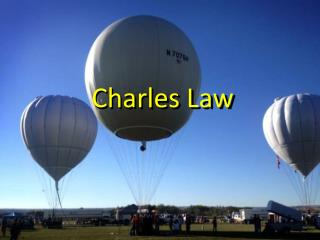DownloadDownload PresentationCharles Law

# Charles Law

Download Presentation## Charles Law

- - - - - - - - - - - - - - - - - - - - - - - - - - - E N D - - - - - - - - - - - - - - - - - - - - - - - - - - -
##### Presentation Transcript

1. Charles Law

2. Charles’s Law • Jacques Charles determined the relationship between temperature and volume of a gas. • He measured the volume of air at different temperatures, and observed a pattern of behavior which led to his mathematical law. • During his experiments pressure of the system and amount of gas were held constant.

3. Volume of balloon at room temperature Volume of balloon at 5°C

4. Volume Temp How does Temperature and Volume of gases relate graphically? V/T = k Pressure, # of particles remain constant

5. Temperature (T) • The temperature of a gas is generally measured with a thermometerin Celsius. • All calculations involving gases should be made after converting the Celsius to Kelvin temperature. • Kelvin = C° + 273

6. V1 V2 = T1 T2 Charles’s Mathematical Law: What if we had a change in conditions? since V/T = k Eg: A gas has a volume of 3.0 L at 127°C. What is its volume at 227 °C?

7. Eg: A gas has a volume of 3.0 L at 127°C. What is its volume at 227 °C? • determine which variables you have: • T1 = 127°C + 273 = 400K • V1 = 3.0 L • T2 = 227°C + 273 = 5ooK • V2 = ?

8. 3.0L V2 = 400K 500K 2) Plug in the variables: 3) Cross multiply and chug (500K)(3.0L) = V2 (400K) V2 = 3.8L